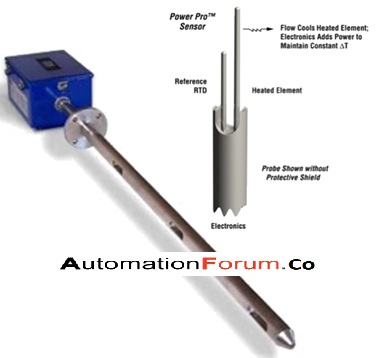# What is mass flow meter? What are the types of mass flow meter#### What is mass flow meter and what is mass flow measurement

A mass-flow meter measures the mass flow rate of the fluid through the pipe, and it won’t measure the volume. The mass flow rate is the mass of fluid that travels past a fixed point per unit time. By using this flow meter we can determine the mass of the substance that travels through the surface per unit time. This flow meter measures the mass from the density of the substance, the cross-sectional area through which the substance is flowing, and its velocity. This type of flow meter is very accurate and it is used to measure the flow of gases and other fluids. Coriolis and thermal flow meters are two types of a mass flow meter. A mass flow meter is required for the accurate measurement of the mass related processes such as chemical reaction, heat transfer, etc.

#### What are the types of mass flow meter and how do they operate

A mass flow meter measures the mass of the substance per unit time that travels through the tube. A volumetric flow rate is the mass flow rate divided by the fluid density. The density of the fluid could vary with temperature, pressure, and composition. There are two types of mass flow meter they are thermal and Coriolis flowmeter

Thermal flow meter

The thermal flow meter measures the mass of the fluid flow with the help of a thermistor, so this meter uses a thermistor that has only one sensor tip and it would be inserted into the flow stream. So it won’t get clogged and can also measure very low flow rates. This flow meter works on the principle of thermal conductivity. This meter will introduce heat into the flow stream and it measures the heat dissipation amount. The thermal mass flow meter is independent of the density, pressure, and viscosity of the fluid.

It can be used to measure the mass flow rate of liquids and gases. So the thermistor will be mounted inside a pipe when the fluid passes it carries the heat away from the thermistor. If the flow rate is high then the thermistor will become cool very fast and increases its resistance. In order to get an accurate value, a second thermistor head can be used.

#### Types of thermal mass flow meter

Constant temperature difference

In this type it uses two temperature sensors, a heated sensor and the other one is to measure the gas temperature and the mass flow rate is determined based on the electrical power needed to keep the temperature difference between the sensors.

Constant power

In this type, constant power is used in the heater. It would measure the temperature difference between the heated sensor and the reference sensor. The mass flow is measured according to the difference between the temperature of the heated sensor and the temperature of the flow stream.

How to install a thermal mass flow meter

The meter must be installed in a way that, it must be full of liquid so that the air won’t be trapped inside the tube. It must not be installed where the flow is downward in a vertical pipe.

What are the advantages of thermal mass flow meter

• It can measure the mass flow directly without using pressure and temperature inputs
• It can give accurate measurement at very low flow rates
• Pressure drop is less
• It is economical
• Maintenance-free
• There is no need for pressure and temperature compensation
• It can be easily installed

What are the applications of thermal mass flow meter

• Oil and gas
• Power
• Chemical
• Water and waste
• Metals and mining
• Food and beverage
• Pharmaceuticals
• Textile industry

Coriolis meter

This device also measures the mass of the liquid and this meter is U shaped. The fluid will flow through a U shaped tube. The tube is made to vibrate and this vibration will be perpendicular to the fluid flow. Because of the energy and velocity of the fluid, it resists the vibrating motion of the tube, and that makes it twist sideways. The degree of deflection is linearly and directly proportional to the mass of the fluid that passes through the tube. The amount of twist is measured by the magnetic position sensors. The output from each sensor is conditioned to standard signals before it is sent to the control equipment. The major advantage of a Coriolis flowmeter is its high accurate mass flow measurement and it is not dependent on the fluid properties.